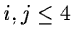Next: Rebinning Up: FEROS Previous: Flat-fielding

Wavelength calibration

The wavelength calibration uses the ThArNe calibration frames. The orders are first extracted and then searched for emission lines. This is done with the command SEARCH/FEROS. Wavelengths are assigned to these lines iteratively using a catalog of wavelengths and starting with a preliminary dispersion relation. A global dispersion relation for the whole wavelength rane is used for fitting the line positions as a function of y-position and order number. The global formula used is of the form(29.1)

where x and m are the position along the dispersion axis and the order number, respectively, and aij are the coefficients to be fitted. For the order of the polynomialis sufficient for FEROS. Most of the higher terms are set to zero, so that only 15 free parameters are used. With this formula we can reach residuals of the order of 3mÅ rms over the full wavelength range. The wavelength calibration is done with the command CALIB/FEROS.

Petra Nass
1999-06-15